# Merge K Sorted Arrays

## Introduction

This blog will cover how to merge ‘K’ sorted arrays. Let’s start by looking at the problem statement. There are K Sorted Arrays, each of size N, and you have to merge all these arrays to make one sorted array and return the result.

Let’s understand the problem with an example. We are using ‘K’ as 3 in this problem, and we have to return one sorted array after merging all these arrays -

Input -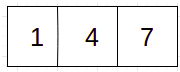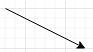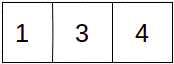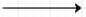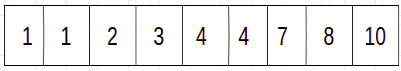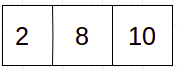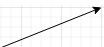## Brute Force Approach

Step 1. Create a resultant array of size N * K.

Step 2. Iterate all the arrays from start to end and insert all the elements in the resultant array.

Step 3.  Sort and print the resultant array.

Java Solution

### Better Approach

In this approach, we merge arrays in pairs. After the first iteration of merging, we have K/2 arrays. After the second merge, we will have K/4 arrays and so on. We will use recursion to implement this approach for the merge K sorted arrays problem.

Step 1. Create a function that takes K arrays and returns the resultant array.

Step 2. Implement base condition: if the value of 'K' is 1 then return the array. If ‘K’ contains two, then merge the two arrays and return the array.

Step 3. If the value of K > 2, then divide the group of 'K' elements into equal half and recursively call the function for each of the halves.

Step 4. Print the resultant array.

Java Solution for Merge K sorted Arrays

Complexity Analysis of merge K sorted arrays problem:

• Time Complexity: O(N * K * log K) where ‘N’ is the size of arrays and ‘K’ is the total number of sorted arrays. Total levels are log(K) and each level is doing N * K work, so complexity is O(N * K * log K).

• Space Complexity: O(N * K * log k) where ‘N’ is the size of arrays and ‘K’ is the total number of sorted arrays. O(N * K) space is being used at each level and total levels are log(K) which makes it O(N * K * log K).

## Efficient Approach for Merge K sorted Arrays

Min Heap can be used to handle this merge K sorted arrays problem but first, let's understand what MinHeap is -

A Min-Heap is a complete binary tree, or we can say a binary tree in which the value contained by each internal node is smaller than or equal to the values in the children of that node. We can store a heap in an array. If a specific node is stored at index ‘K’ of the array, then the left child of that node is stored at index 2 * K + 1, and the right child of that node is stored at index 2 * K + 2.

This solution has the same time complexity, which is O(N * K * log K). But it works much better. This algorithm starts with creating a MinHeap and inserting the first element of all the 'K' arrays, then removing the root element of Min Heap and putting it in the resultant array named 'res' in the code and inserting the next element from the array of the removed elements. To get the result, we have to execute this step until there is no element left in Min Heap.

Algorithm for the efficient approach

Step 1. Create a min-heap following the definition mentioned above and insert the first element of 'K' arrays.

Step 2. Iterate until the MinHeap size > 0.

Step 3. Get the top element of the MinHeap and this top element is the minimum element and prints the element.

Step 4. Insert the next element from the same respective array from which the element was removed.

Step 5. If the array doesn’t have any more elements, then replace root with infinite. After replacing the root, heapify the tree.

Example:

Input:

Java Solution

1. What is a Sorted Array?

An array is a collection of elements stored in continuous storage blocks in the memory. Sorting in programming refers to placing the elements of a data structure in a specific and meaningful manner. Sorting is an essential part of data processing. Efficient sorting algorithms are crucial so that we can perform operations that require sorted input optimally. Therefore, the array having elements in either increasing or decreasing order is known as a Sorted Array.

2.  How are we handling the case of duplicate elements?

Let’s consider the Min Heap approach, we are removing minimum elements in every iteration so if duplicate elements are present in min-heap, the getMin() function will remove them one by one which will help to handle the case of duplicate elements.

3.  What is Min Heap?

A Min-Heap is a complete binary tree, or we can just say a binary tree (which means each node can have a maximum of 2 children) in which each internal node has a value that is smaller than or equal to the values in the children node. We can store a heap in an array. If a node is stored at index ‘K’, then its left child is stored at index 2 * K + 1 and its right child at index 2 * K + 2.

## Key Takeaways

In this article, we discussed how to merge K Sorted Arrays and discussed the different approaches to tackle the merge K sorted arrays problem along with their complexities. We also learned about Min Heap and how we leveraged it to improve the complexity of the solution. You can also refer to our DSA course in C++  and Java for more details in Heaps and Priority Queues.